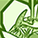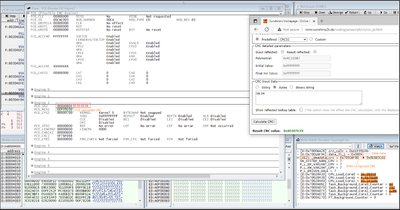Announcements

# AURIX™ Forum DiscussionsLevel 3
Level 3# Calculate CRC-32 for 16bit data using FCE

Hi ,
Background : We transfer 12bit Single Channel ADC data over DMA and want to compute CRC using our SW and compare it with the checksum computed by the DMA. For our SW computation we use FCE to calculate CRC.
Problem :
Lets say the data we transferred by DMA = 0x804
DMA computed checksum = 0x8E6D5CEE

When I use FCE to compute the CRC the Input data is always taken in 32 bit format i.e 0x00 0x00 0x08 0x04.
And the CRC I get for this is 0xEEF0910D

Both the CRC's are correct the only difference is the input data format.
Question : Is it possible to use FCE to calculate CRC-32 for a 16 bit data ? If so could you please assist me on this.
Screenshot :Note : Changed DMA data transfer width to 32 bit and CRC computation is okay. But as we use only one ADC channel that other half contains some garbage data.

Thanks,
Kumaresh
2 RepliesModeratorModerator# Re: Calculate CRC-32 for 16bit data using FCE

Hi,

In FCE, the bit width of each word should be in terms of KERNEL polynomial width.
You would need to pad the 16-bit data.

https://community.infineon.com/t5/AURIX/Calculated-CRC-from-FCE-on-the-DMA-data-being-transferred-do...

Regards,
Aiswarya.Level 3
Level 3# Re: Calculate CRC-32 for 16bit data using FCE

Hi, If I pad other 16 bits to the data which I need to calculate CRC using FCE then it will be different by the CRC calculated by DMA.  Does the padded bits also considered in CRC calculation? From my testing I see that the CRC is computed with padded bits. So thought to confirm with you